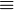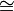# Equality (mathematics) facts for kids

Kids Encyclopedia Facts

In mathematics, two things are equal if and only if they are exactly the same in every way. That is, they have the same (mathematical) value and the same mathematical properties. Mathematicians use the equals sign (=) to say this. This defines a binary relation, equality. The statement "x = y" means that x and y are equal.

Equivalence in a more general sense is provided by the construction of an equivalence relation between two mathematical objects, that is, two mathematical objects are equivalent if they are related by this relation. In which case, the equivalence is often represented using the symbols$\sim$ or$\equiv$.

A statement that two expressions denote equal quantities is an equation (or an equality). Equations are equal. Inequalities are unequal.

An equality is also a transitive relation. This means that if one object is equal to a second object, and the second object is equal to a third object, then the first object is equal to the third object as well.

Since a predicate is a way of describing something that is true, another way to say this is that if one thing that is true about a variable is not true about the other variable, then they are not equal as far as mathematical logic is concerned: two things are only equal if anything that is true about one has to be true about the other.

In geometry, the word congruence is often preferred. Numbers are equal, geometrical objects are congruent. Two shapes are congruent if one can be moved or rotated so that it fits exactly where the other one is. If shrinking or enlarging one of the two objects is needed, then they are not congruent. They are called similar instead. The congruence relation is often represented by the symbol$\cong$, while the similarity relation is represented by the symbol$\sim$.

In computer science, usually, the mathematical definition is used. Very often, the comparison is written == (and the assignment, the act of giving a value, is written as =, or :=). In object-oriented languages, or languages which have pointers, there is an additional problem. Those languages contain references (which are in fact pointers). If two such references do not reference exactly the same object, then they are different and a == b will be false in this case.

For this reason, many such languages have introduced another operator (in Java, this method is called equals). This operator compares the actual values of the objects—not where the variables that reference them point to.

In social sciences, two people are equal if many of the same things are true about them. For example. two people who have the same amount of education and money, and who are of the same age usually think of each other as equals. Another name for a person who is equal to another person is a peer.

## Related pagesEquality (mathematics) Facts for Kids. Kiddle Encyclopedia.